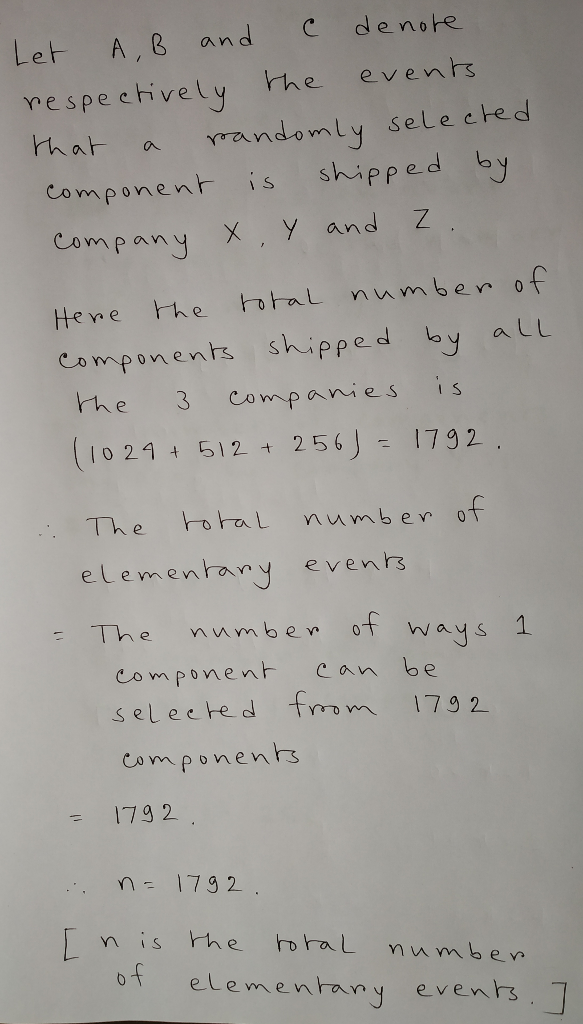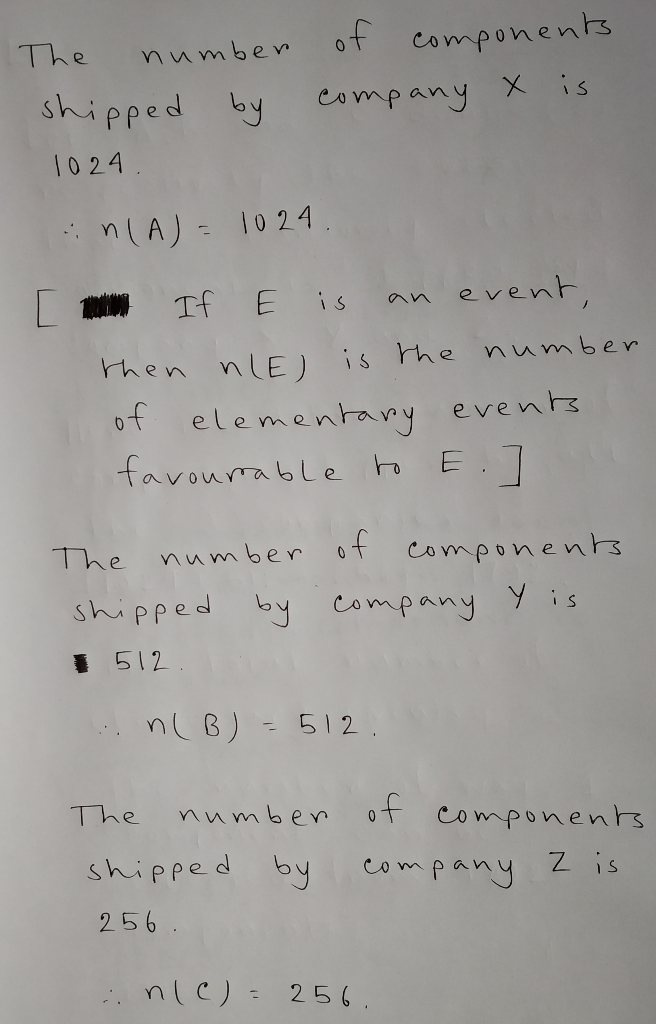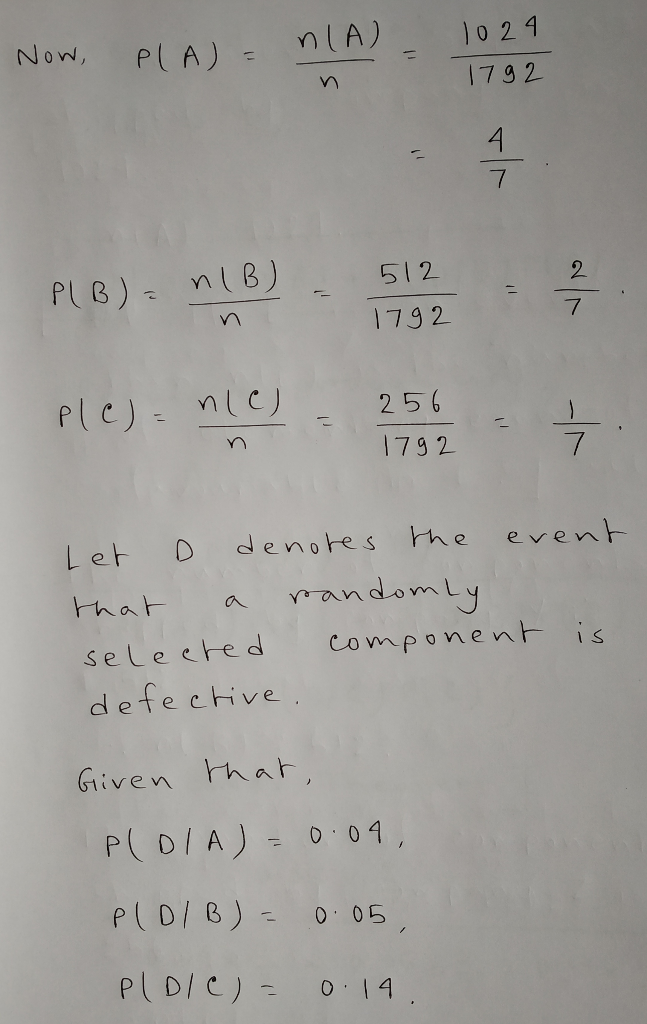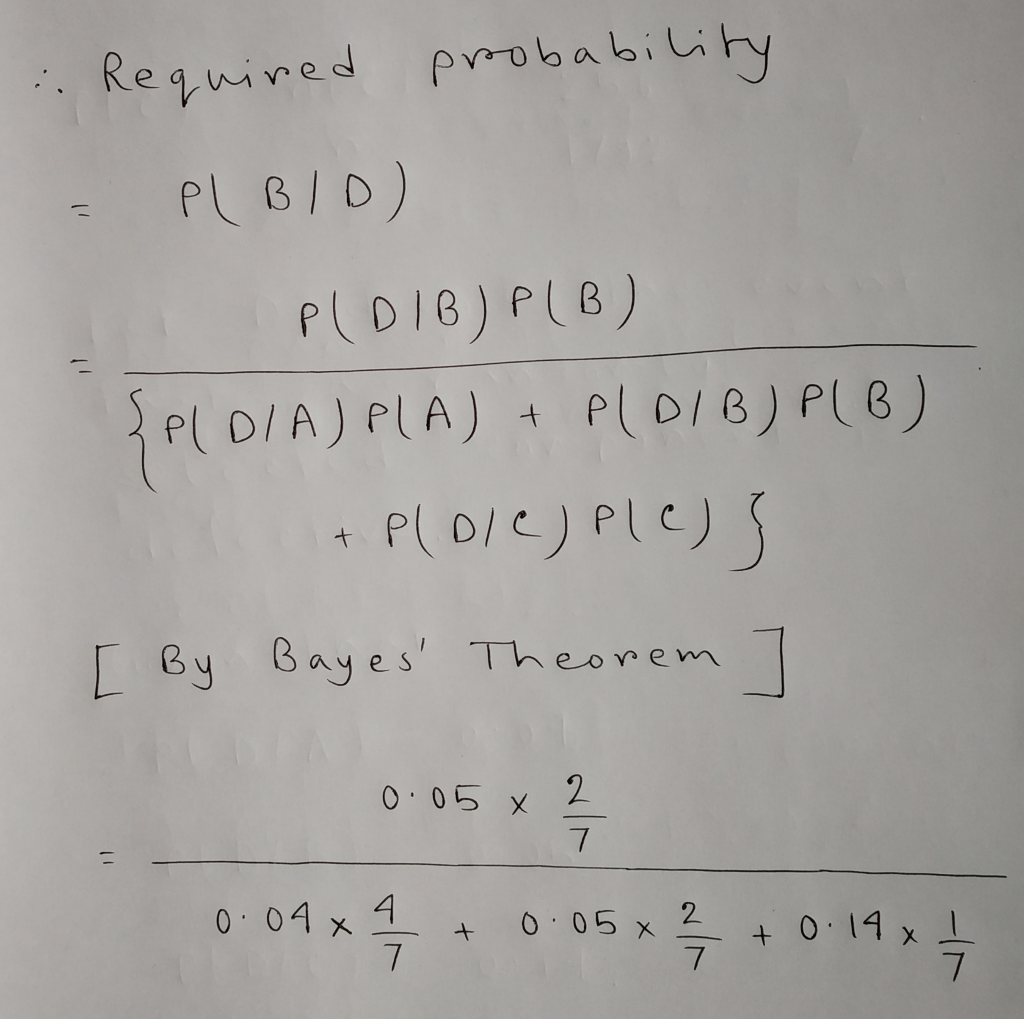In: Math

# Companies X, Y and Z all manufacture a specific component required to make a touch screen...

Companies X, Y and Z all manufacture a specific component required to make a touch screen tablet. Of these three companies, X shipped 1024 components, Y shipped 512 and Z shipped 256. The percentage of defective components produces by each company is 4%, 5% and 14% for X, Y and Z, respectively. What is the probability that a given defective component came from Company Y?

## Solutions

##### Expert Solution## Related Solutions

##### What are (a) the x component, (b) the y component, and (c) the z component of...
What are (a) the x component, (b) the y component, and (c) the z component of r Overscript right-arrow EndScripts equals a Overscript right-arrow EndScripts minus b Overscript right-arrow EndScripts plus c Overscript right-arrow EndScripts if a Overscript right-arrow EndScripts equals 5.4 i Overscript ̂ EndScripts plus 1.9 j Overscript ̂ EndScripts minus 3.6 k Overscript ̂ EndScripts , b Overscript right-arrow EndScripts equals negative 4.1 i Overscript ̂ EndScripts plus 5.4 j Overscript ̂ EndScripts plus 3.7 k Overscript...
##### Describe the level surfaces of G(x,y, z) = 1 – y^2 – z. Make sure to...
Describe the level surfaces of G(x,y, z) = 1 – y^2 – z. Make sure to provide all the following: their equations, types, plots, and a short word description of their geometric shapes.
##### The curried version of let f (x,y,z) = (x,(y,z)) is let f (x,(y,z)) = (x,(y,z)) Just...
The curried version of let f (x,y,z) = (x,(y,z)) is let f (x,(y,z)) = (x,(y,z)) Just f (because f is already curried) let f x y z = (x,(y,z)) let f x y z = x (y z)
##### Let X, Y ⊂ Z and x, y ∈ Z Let A = (X\{x}) ∪ {x}....
Let X, Y ⊂ Z and x, y ∈ Z Let A = (X\{x}) ∪ {x}. a) Prove or disprove: A ⊆ X b) Prove or disprove: X ⊆ A c) Prove or disprove: P(X ∪ Y ) ⊆ P(X) ∪ P(Y ) ∪ P(X ∩ Y ) d) Prove or disprove: P(X) ∪ P(Y ) ∪ P(X ∩ Y ) ⊆ P(X ∪ Y )
##### If X, Y and Z are three arbitrary vectors, prove these identities: a. (X×Y).Z = X.(Y×Z)...
If X, Y and Z are three arbitrary vectors, prove these identities: a. (X×Y).Z = X.(Y×Z) b. X×(Y×Z) = (X.Z)Y – (X.Y)Z c. X.(Y×Z) = -Y.(X×Z)
##### For each of the formulas below, state whether it is true or false. a) pX,Y,Z(x,y,z)=pY(y)pZ∣Y(z∣y)pX∣Y,Z(x∣y,z)   ...
For each of the formulas below, state whether it is true or false. a) pX,Y,Z(x,y,z)=pY(y)pZ∣Y(z∣y)pX∣Y,Z(x∣y,z)       Select an option         True         False    b) pX,Y∣Z(x,y∣z)=pX(x)pY∣Z(y∣z)       Select an option         True         False    c) pX,Y∣Z(x,y∣z)=pX∣Z(x∣z)pY∣X,Z(y∣x,z)       Select an option         True         False    d) ∑xpX,Y∣Z(x,y∣z)=1       Select an option         True         False    e) ∑x∑ypX,Y∣Z(x,y∣z)=1       Select an option         True   ...
##### Find ??, ?? and ?? of F(x, y, z) = tan(x+y) + tan(y+z) – 1
Find ??, ?? and ?? of F(x, y, z) = tan(x+y) + tan(y+z) – 1
##### Three materials X, Y, and Z are required to produce two products A and B. The...
Three materials X, Y, and Z are required to produce two products A and B. The profit function of each product is nonlinear. The total profit function for Product A is 80A - A2 and the total profit function for Product B is 72B - 0.8B2. Thus, the total profit for producing A and B together is 80A - A2 + 72B - 0.8B2. (These two profit functions are independent.) a. Use the Nonlinear Solver (GRG Nonlinear) to solve the...
##### Given function f(x,y,z)=x^(2)+2*y^(2)+z^(2), subject to two constraints x+y+z=6 and x-2*y+z=0. find the extreme value of f(x,y,z)...
Given function f(x,y,z)=x^(2)+2*y^(2)+z^(2), subject to two constraints x+y+z=6 and x-2*y+z=0. find the extreme value of f(x,y,z) and determine whether it is maximum of minimum.
##### *(1)(a) Find a formula for the intersection of a cone {(x,y,z): x^2+y^2=z^2} with a plane {(x,y,z):...
*(1)(a) Find a formula for the intersection of a cone {(x,y,z): x^2+y^2=z^2} with a plane {(x,y,z): z=c}. (b) Find a formula for the intersection of a cone {(x,y,z): x^2+y^2=z^2} with a plane {(x,y,z): x=a}. (c) Find a formula for the intersection of a cone {(x,y,z): x^2+y^2=z^2} with a plane {(x,y,z): y=b}. *(2) Find a formula for the intersection of a cone {(x,y,z): x^2+y^2=z^2} with a plane {(x,y,z): z=kx+b} assuming both b and k are positive. (a) For what value of...